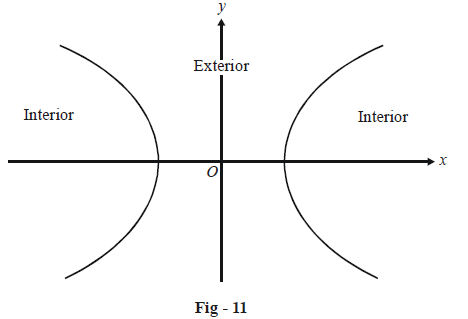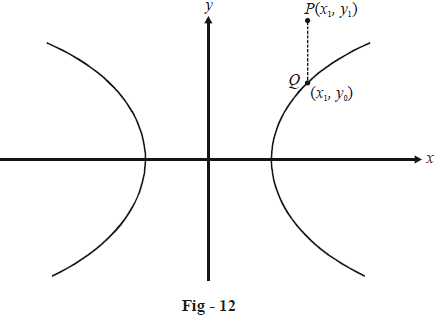# Basic Examples on Hyperbolas Set 3

Go back to  'Hyperbola'

Example - 7

Find the equation of the chord joining the points $$P({\theta _1})$$ and $$Q({\theta _2})$$ on the hyperbola \begin{align}\frac{{{x^2}}}{{{a^2}}} - \frac{{{y^2}}}{{{b^2}}} = 1.\end{align}

Solution : The two points P and Q have the coordinates $$(a\sec {\theta _1},\,\,b\tan {\theta _1})$$and $$(a\sec {\theta _2},\,\,b\tan {\theta _2})$$ respectively. Using the two-point form, the equation of PQ is

\begin{align}&\,\,\,\,\,\,\,\,\,\,\,\frac{{y - b\tan {\theta _1}}}{{x - a\sec {\theta _1}}} = \frac{{b\tan {\theta _1} - b\tan {\theta _2}}}{{a\sec {\theta _1} - a\sec {\theta _2}}}\\\\ &\Rightarrow \frac{{y - b\tan {\theta _1}}}{{x - a\sec {\theta _1}}} = \frac{b}{a}\left( {\frac{{\sin {\theta _1}\cos {\theta _2} - \cos {\theta _1}\sin {\theta _2}}}{{\cos {\theta _2} - \cos {\theta _1}}}} \right)\end{align}

Using simple trigonometric relations, this can be written as

$\boxed{\frac{x}{a}\cos \left( {\frac{{{\theta _1} - {\theta _2}}}{2}} \right) - \frac{y}{b}\sin \left( {\frac{{{\theta _1} + {\theta _2}}}{2}} \right) = \cos \left( {\frac{{{\theta _1} + {\theta _2}}}{2}} \right)}$

This is a useful general equation; it gives us the line joining any two points on the hyperbola. It would be advantageous to remember this equation.

Example - 8

When will a chord joining two points $${\theta _1}{\rm{\; and \;}}{\theta _2}$$ on the hyperbola \begin{align}\frac{{{x^2}}}{{{a^2}}} - \frac{{{y^2}}}{{{b^2}}} = 1\end{align} pass through its focus (ae,0)?

Solution: We simply substitute $$x \to ae$$ and $$y \to 0$$ in the general equation for the chord we obtained above. Thus, we obtain

\begin{align}&\,\,\,\,\,\,\,\,\,\,\,\,\,\,\,\,\,\,e\cos \left( {\frac{{{\theta _1} - {\theta _2}}}{2}} \right) = \cos \left( {\frac{{{\theta _1} + {\theta _2}}}{2}} \right)\\ &\Rightarrow \quad\frac{{\cos \left( \begin{align}{\frac{{{\theta _1} - {\theta _2}}}{2}}\end{align} \right)}}{{\cos \left(\begin{align} {\frac{{{\theta _1} + {\theta _2}}}{2}}\end{align} \right)}} = \frac{1}{e}\end{align}

This is the required condition that must be satisfied. To write it more simply, we apply C and D to obtain.

\begin{align}&\frac{\begin{align}{\cos \left( {\frac{{{\theta _1} - {\theta _2}}}{2}} \right) + \cos \left( {\frac{{{\theta _1} + {\theta _2}}}{2}} \right)}\end{align}}{\begin{align}{\cos \left( {\frac{{{\theta _1} - {\theta _2}}}{2}} \right) - \cos \left( {\frac{{{\theta _1} + {\theta _2}}}{2}} \right)}\end{align}} = \frac{{1 + e}}{{1 - e}}\end{align}

This finally yields

$\tan \left( {\frac{{{\theta _1}}}{2}} \right)\tan \left( {\frac{{{\theta _2}}}{2}} \right) = \frac{{1 - e}}{{1 + e}}$

Example - 9

Let us define the exterior and interior regions of a hyperbola \begin{align}S(x,\,\,y):\frac{{{x^2}}}{{{a^2}}} - \frac{{{y^2}}}{{{b^2}}} = 1\end{align} as follows:How can we decide whether a point $$P({x_1},{y_1})$$ lies in the interior or exterior region of the hyperbola?

Solution: Let us consider $$P({x_1},{y_1})$$ lying outside the hyperbola as shown below:We have dropped a perpendicular from P onto the x-axis intersecting the hyperbola at$$Q({x_1},{y_0}).$$ Since Q lies on the hyperbola, we have

\begin{align}&\!\;\;\,\,\,\,\,\,\,\,\,\,\,\,\,\,\,\,\,\,\frac{{x_1^2}}{{{a^2}}} - \frac{{y_0^2}}{{{b^2}}} = 1\\ &\Rightarrow \qquad y_0^2 = {b^2}\left( {\frac{{x_1^2}}{{{a^2}}} - 1} \right)\end{align}

Since $${y_1} > {y_0},$$ we have

\begin{align}&\;\;\;\;\;\;\,\,\,\,\,\,\,\,\,\,\,\,\,\,\,\,y_1^2 > {b^2}\left( {\frac{{x_1^2}}{{{a^2}}} - 1} \right)\\ &\Rightarrow \qquad \frac{{x_1^2}}{{{a^2}}} - \frac{{y_1^2}}{{{b^2}}} - 1 < 0\\\\ &\Rightarrow \qquad S\left( {{x_1},\,\,{y_1}} \right) < 0\end{align}

Convince yourself that for any point $$P({x_1},\,\,{y_1})$$ lying exterior to the hyperbola, this condition must be satisfied. Similarly for any point $$P({x_1},\,\,{y_1})$$ lying in the interior region, we must have $$S({x_1},{y_1}) > 0.$$

We can summarize this discussion as:

$$S({x_1}\;,\;{y_1}) > 0\quad \Rightarrow \quad P({x_1}\;,\;{y_1})\;\;\text{ lies inside the hyperbola.}$$

$$S({x_1}\;,\;{y_1}) = 0 \quad \Rightarrow \quad P({x_1}\;,\;{y_1}) \;\;\text{lies on the hyperbola}$$

$$S({x_1}\;,\;{y_1}) < 0 \quad \Rightarrow \quad P({x_1}\;,\;{y_1}) \;\;\text{lies outside the hyperbola}$$.

Example - 10

Find the condition that must be satisfied if the line $$y = mx + c$$ is to intersect the hyperbola \begin{align}\frac{{{x^2}}}{{{a^2}}} - \frac{{{y^2}}}{{{b^2}}} = 1\end{align} in two distinct points. As a corollary, when will this line be a tangent to the hyperbola ?

Solution: The requisite condition can be obtained by solving simultaneously the equations of the line and the hyperbola.

\begin{align}&\;\;\,\,\,\,\,\,\,\,\,\,\,\,\,\,\,\,\,y = mx + c\\ \\\,\,\,\,\,\,\,\,\,\,\,\,\,\,\,\,\,&\qquad\quad\;\frac{{{x^2}}}{{{a^2}}} - \frac{{{y^2}}}{{{b^2}}} = 1\\ \\&\Rightarrow \qquad \frac{{{x^2}}}{{{a^2}}} - \frac{{{{(mx + c)}^2}}}{{{b^2}}} = 1\\ \\&\Rightarrow \qquad ({a^2}{m^2} - {b^2}){x^2} + 2{a^2}cmx + {a^2}({c^2} + {b^2}) = 0\end{align}

This quadratic will have two distinct roots if its discriminant is positive, i.e., if

\begin{align}&\;\;\;\,\,\,\,\,\,\,\,\,\,\,\,\,\,\,\,\,4{a^4}{m^2}{c^2} > 4{a^2}({b^2} + {c^2})({a^2}{m^2} - {b^2})\\\\ &\Rightarrow \qquad {a^2}{m^2}{c^2} > {a^2}{m^2}{b^2} - {b^4} + {a^2}{m^2}{c^2} - {b^2}{c^2}\\\\ &\Rightarrow \qquad {c^2} > {a^2}{m^2} - {b^2}\end{align}

If this condition is satisfied, the line will intersect the hyperbola in two distinct points. It is clear that the line will be a tangent if

\begin{align}&\;\;\;\,\,\,\,\,\,\,\,\,\,\,\,\,\,\,\,\,{c^2} = {a^2}{m^2} - {b^2}\\\\ &\Rightarrow \qquad {c^2} = \pm \sqrt {{a^2}{m^2} - {b^2}}\end{align}

Thus, we can conclude that any line of the form $$y = mx \pm \sqrt {{a^2}{m^2} - {b^2}}$$ will always be a tangent to the hyperbola \begin{align}\frac{{{x^2}}}{{{a^2}}} - \frac{{{y^2}}}{{{b^2}}} = 1,\end{align} whatever be the value of m.

As an exercise prove that the coordinates of the point of contact of this tangent(s) will be

\begin{align}&\left( { \pm \frac{{{a^2}m}}{{\sqrt {{a^2}{m^2} - {b^2}} }},\,\, \pm \frac{{{b^2}}}{{\sqrt {{a^2}{m^2} - {b^2}} }}} \right)\end{align}

We discuss about tangents in more detail in the next section.

## TRY YOURSELF - I

Q. 1 Find the equation of the hyperbola with the following parameters:

 $$\text{Focus}\quad \quad \quad \quad \,\,\,\,:$$ $$(1,2)$$ $$\text{Directrix}\quad \quad \quad \,\,:$$ $$2x + y - 1 = 0$$ $$\text{Eccentricity}\quad \quad \,:$$ $$\sqrt 3$$

Q. 2 Find the equation of the hyperbola with foci at $$(0,\, \pm \sqrt {10} )$$ and which passes through (2, 3).

Q. 3 Find the equation of the hyperbola whose conjugate axis is of length 5 and the distance between whose foci is 13.

Q. 4 Prove that the locus of the point of intersection of the lines

$\begin{array}{l}\sqrt 3 x - y - 4\sqrt 3 \lambda = 0\\\sqrt 3 \lambda x + \lambda y - 4\sqrt 3 = 0\end{array}$

for different values of $$\lambda$$ is a hyperbola. What is the eccentricity of this hyperbola?

Q. 5 For what value of k does the line $$3x - y + k = 0$$ touch the hyperbola \begin{align}\frac{{{x^2}}}{5} - \frac{{{y^2}}}{9} = 1\,\,?\end{align}

Download SOLVED Practice Questions of Basic Examples on Hyperbolas Set 3 for FREE
Hyperbolas
grade 11 | Questions Set 1
Hyperbolas
grade 11 | Answers Set 1
Hyperbolas
grade 11 | Questions Set 2
Hyperbolas
grade 11 | Answers Set 2
Learn from the best math teachers and top your exams

• Live one on one classroom and doubt clearing
• Practice worksheets in and after class for conceptual clarity
• Personalized curriculum to keep up with school